### Home > CALC > Chapter 4 > Lesson 4.4.1 > Problem4-130

4-130.
1. Evaluate. Homework Help ✎

1.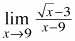2.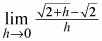3.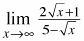4.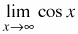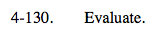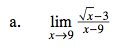You could multiply the numerator and denominator by the conjugate of the numerator.

Or... you could recognize that this is Ana's Definition of the Derivative.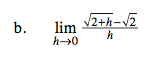This is Hana's Definition of the Derivative.

What is f(x)?
What is a?
What is f '(x)?
The limit = f '(a) =_________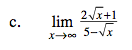This limit is approaching infinity. What is the end behavior? Is there a horizontal asymptote? Compare the highest-power terms in the numerator and denominator. Pay attention to coefficients.

= −2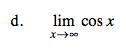Visualize the graph of y = cosx. It oscillates as x→∞.